Organic Chemistry : Help with Acid-Base Reactions

Example Questions

Example Question #1 : Help With Acid Base Reactions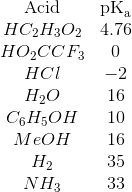For which of the following acid-base reactions will the equilibrium lie on the left side?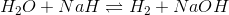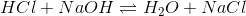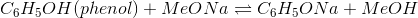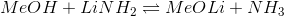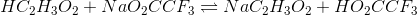Explanation:

The pKa value indicates how strong an acid is, and acid strength increases as pKa decreases. The side of a reaction with a lower pKa is going to dissociate more, pushing the equilibrium over to the other side. The equilibrium will thus lie on the side with the HIGHER pKa.Since the pKa of acetic acid (4.76) is higher than the pKa of trifluoroacetic acid (0), the reaction will shift to the left to reach equilibrium.

Example Question #75 : Organic Concepts

A carboxylic acid has a pKa of 5. At a pH of 8, what is the ratio of salt to acid?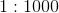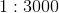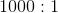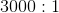Explanation:

Use the Henderson Hasselbalch equation: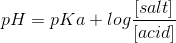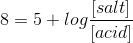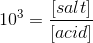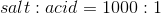Example Question #142 : Organic Chemistry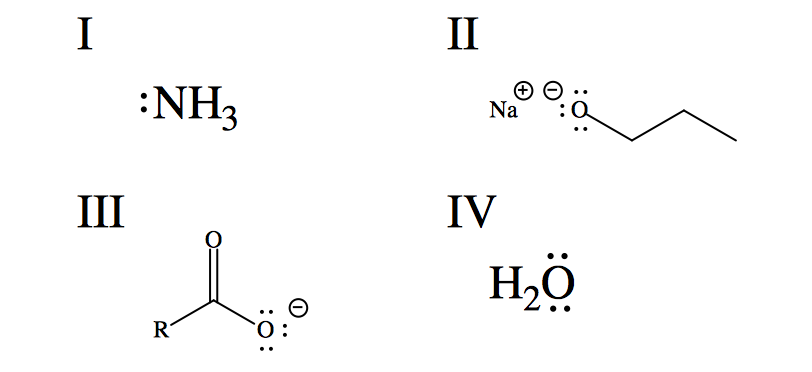List the given compounds in order of decreasing basicity.

II, III, I, IV

IV, I, III, II

II, I, III, IV

II, III, IV, I

III, II, IV, I

II, I, III, IV

Explanation:

An easy way to consider relative base strengths is to consider the strength of the compounds' conjugate acids.The stronger the conjugate acid, the weaker the base. Water (compound IV) is the least basic of the compounds because its conjugate acid,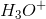is the strongest of the given compounds' conjugate acids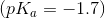. Carboxylate ions (compound III) are highly stabilized by resonance and predominate at neutral pH. The conjugate carboxylic acids readily donate protons (acetic acid: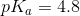). Ammonia (compound I) has considerable basicity; binding a fourth hydrogen produces ammonium ion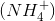, which predominates at neutral pH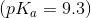Sodium propoxide (compound II) is a strong base, bearing a full negative charge on its oxygen. Its conjugate acid, 1-propanol, is a rather weak acid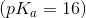. Since its conjugate acid is the weakest (highest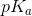), sodium propoxide is the strongest base. Based on the previous observations the correct ordering of the compounds is: II, I, III, IV.

Example Question #77 : Organic Concepts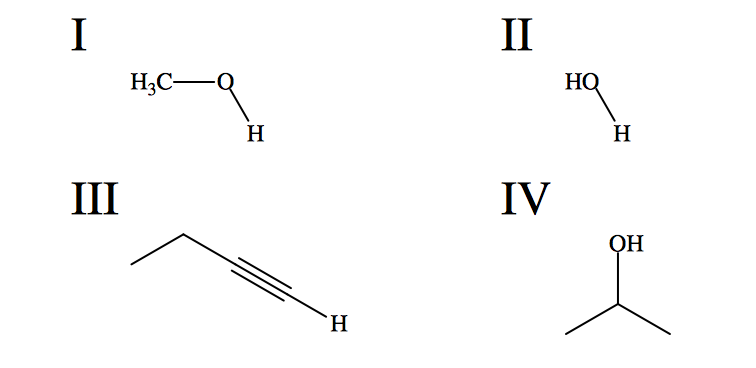Rank these weak acids by decreasing(of the expressed hydrogens).

IV, III, I, II

III, IV, II, I

II, III, I, IV

III, IV, I, II

IV, I, II, III

III, IV, II, I

Explanation:

The governing principle regarding the prediction ofvalues (relative to other compounds) is to assess the stability of the product formed by the release of a proton. The release of the alkyne hydrogen in compound III results in a carbanion, a highly unstable species, so it is expected that this compound is the least acidic and has the highest. Intuition serves well in this instance and we see that hydrogens bound to a triple bond have avalue of around 25. The relative stabilities of the remaining compounds may be assessed in the same manner. Compound IV is the second weakest acid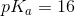because the three methyl groups donate electron density such that if the oxygen is deprotonated, the resulting negative charge is destabilized. Methanol and water have a unique, non-intuitive relationship regarding their relative acidities. One would assume that water should be a stronger acid than other acids bound to alkyl groups (by the reasoning expressed for compound IV). This is the case for all alcohols except methanol, in which the delocalization of charge allowed by the increased molecular size outweighs the destabilization caused by electron donation. Thus methanol is a slightly stronger acid than water. This is evidenced in theirvalues: 15.7 for water and 15.5 for methanol. The correct ordering of the given compounds is: III, IV, II, I.

Example Question #78 : Organic Concepts

A.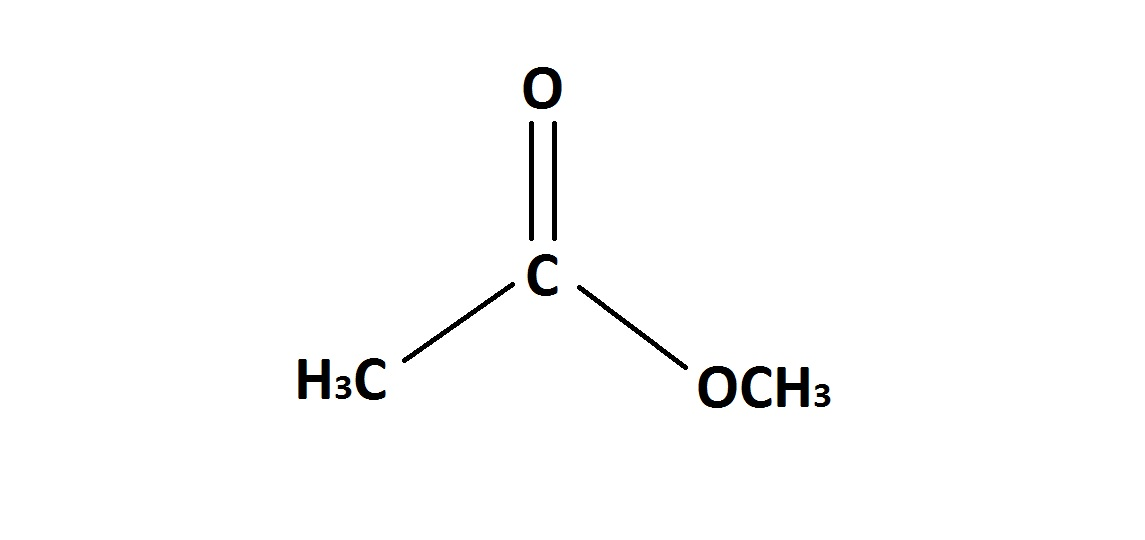B.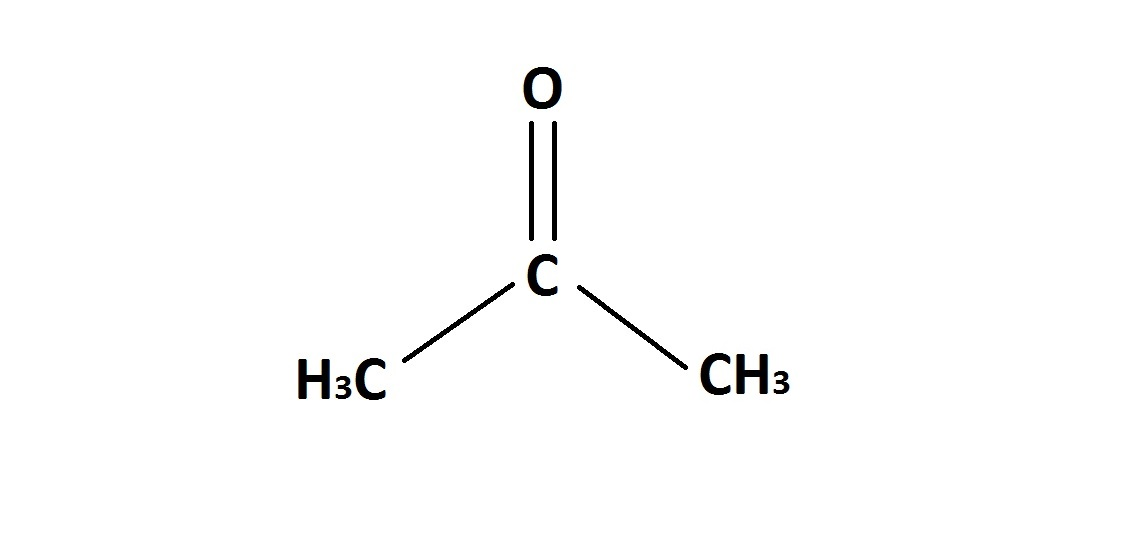Which of the above molecules is expected to have a more acidic alpha-carbon, and why?

Molecule B, because its conjugate base has more stability.

Molecule B, because its conjugate base has less stability.

Molecule A, because its conjugate base has less stability.

Molecule A, because its conjugate base has more stability.

Molecule B, because its conjugate base has more stability.

Explanation:

Molecule B will have a more acidic alpha-carbon because once the alpha proton becomes dissociated, the conjugate base will have relatively more stability than the conjugate base of molecule A.

When the alpha-carbon on molecule A loses it's proton, the conjugate base is not as stable. The reason for this is because the oxygen that is involved in the ester bond can contribute its electrons towards a resonance structure. Therefore, after the alpha-proton is lost, the alpha-carbon will have a negative charge that will be destabilized by the delocalized negative charge of the resonance structures.

Example Question #79 : Organic Concepts

Which of the following is the strongest acid?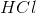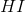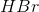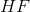Explanation:

From the start, we know we can eliminate answer choicebecause it is the only answer choice that is not a strong acid. Now we have three strong acids, but we have to determine which is strongest. To do so, we take the conjugate base of each strong acid to see which conjugate base is the weakest acid. Remember: weaker conjugate base means a stronger acid.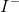is the largest ion of the bunch. Its large size allows it to better stabilize the negative charge and so it is the weakest (most stable) conjugate base. Because the weakest conjugate base leads to the strongest acid,is our correct answer.

Example Question #81 : Organic Concepts

Which of the following sets of bases are listed from most basic to least basic?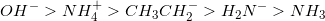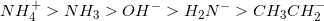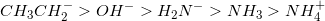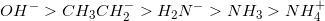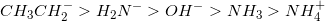Explanation:

The correct ranking from most basic to least basic is:The best bases are negatively charged, and the worst bases are positively charged (acidic). The stronger the base, the weaker (more stable) it's conjugate acid. An alkane is a very stable conjugate acid, which tells us that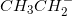is the most basic of the set.

We know based on charge alone, that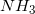is more basic than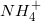but less basic than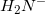or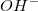.

We know thatis more basicbecause the electronegativity of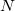is less than that of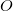. This means the lone pair of electrons onare held less tightly and more likely to pick up a proton.

Example Question #82 : Organic Concepts

Which of the following sets of acids are correctly listed from most to least acidic?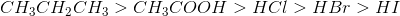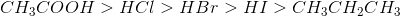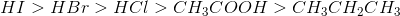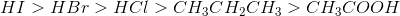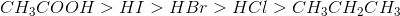Explanation:

Remember that the strongest acids have the weakest conjugate bases.is more acidic thanbecause iodine has a larger atomic radius than bromine.,, andare strong acids and should be at the beginning of the list. Alkanes are not acidic. Acetic acid is a weak acid (pKa =4-5).

Example Question #83 : Organic Concepts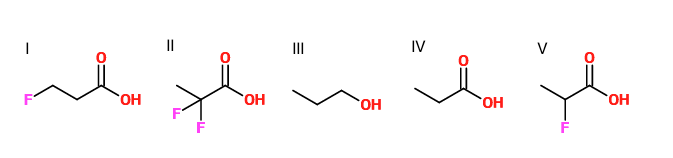Rank the given molecules in order of increasing pKa.

IV, II, V, I, III

II, V, IV, I, III

III, II, V, I, IV

III, I, IV, V, II

I, II, V, IV, III

II, V, IV, I, III

Explanation:

Recall that the stronger an acid, the lower the pKa.

II (two fluorine atoms really close to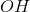has largest inductive effect, so bond is most weakened, and pKa is lowest)

V (one fluorine really close tohas strong inductive effect)

I (one fluorine a little further away fromhas weaker inductive effect)

IV (no inductive effect)

III (alcohols are much less acidic than carboxylic acids, and it has the highest pKa of all)

Example Question #2 : Help With Acid Base Reactions

Which side (left or right) of the following reaction is favored and why?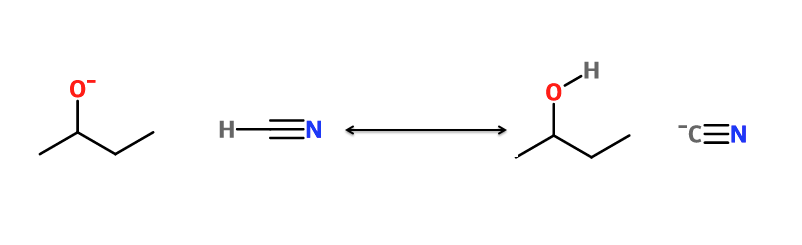Left side, because theof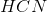is lower than theof 2-butanol.

Right side, because theofis lower than theof 2-butanol.

Right side, because theofis higher than theof 2-butanol.

Left side, because theofis higher than theof 2-butanol.

Right side, because theofis lower than theof 2-butanol.
The side of the reaction that is favored will have the acid with the higher, because the reaction goes (strong acid + strong base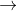weak acid + weak base).